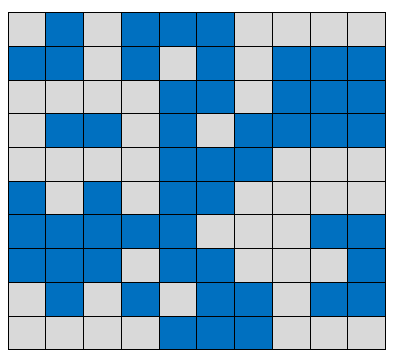# Count the number of islands

Given a binary matrix where 0 represents water and 1 represents land, count the number of islands in it. A island is formed by connected one’s.

For example, consider below image.Above image highlights water in blue and land in grey in a 10 x 10 matrix. There are total five islands present in the above matrix. They are marked by numbers 1-5 in below image.The idea is inspired from finding number of connected components in a graph problem and uses BFS.

The idea is to start BFS from each unprocessed node and increment the island count. Each BFS traversal will mark all cells which make one island as processed. So the problem reduces to finding number of BFS calls.

In each BFS traversal, we start by creating an empty queue. Then we enqueue starting cell and mark it as processed. Then we pop front node from the queue and process all 8 adjacent cells of current cell and enqueue each valid cell which is land. We repeat this process till queue is not empty.

We can find all the possible locations we can move to from the given location by using the array that stores the relative position of movement from any location. For example, if the current location is
(x, y), we can move to (x + row[k], y + col[k]) for 0 <= k <= 7 using below array.

int row[] = { -1, -1, -1, 0, 0, 1, 1, 1 };
int col[] = { -1, 0, 1, -1, 1, -1, 0, 1 };

So, from position (x, y), we can move to:

(x – 1, y – 1)
(x – 1, y)
(x – 1, y + 1)
(x, y – 1)
(x, y + 1)
(x + 1, y – 1)
(x + 1, y)
(x + 1, y + 1)

## C++

Output:

Number of islands are 5

## Java

Output:

Number of islands are 5

Time complexity of above solution is O(MN).

Exercise: Solve above problem using DFS(1 votes, average: 5.00 out of 5)Loading...